International Journal of Materials Science and Applications
Volume 6, Issue 1, January 2017, Pages: 45-53

Theoretical Study of the Spectral Response in Near Infrared of III-V Photodetectors Based on Al, Ga, In, and Sb: The Window Layer Effect

Laboratory of Semiconductors and Solar Energy, Physics Department, Faculty of Science and TechnologyUniversity Cheikh Anta DIOP, Dakar, Senegal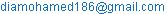(M. Dia)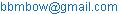(B. Mbow)

Mamadou Dia, Babacar Mbow, EL Hadji Mamadou Keita, Abdoul Aziz Correa, Mamadou Lamine Sow. Theoretical Study of the Spectral Response in Near Infrared of III-V Photodetectors Based on Al, Ga, In, and Sb: The Window Layer Effect. International Journal of Materials Science and Applications. Vol. 6, No. 1, 2017, pp. 45-53. doi: 10.11648/j.ijmsa.20170601.17

Received: December 8, 2016; Accepted: December 20, 2016; Published: January 18, 2017

Abstract: The spectral responses are particularly important for the design of photodetectors operating in the wavelength range between 0,78μm to 2,6μm. To elaborate each device; we purpose a theoretical model to calculate the internal quantum efficiency. The theoretical curves of the internal quantum efficiency are obtained, by simulation to define the geometrical and photoelectric parameters, of the photodetectors devices. The modelling of the various devices allows us to analyze the situation at surface and in volume of each model. The results and the theoretical models, of the heterostructures based on Ga1-xInxSb, Ga1-yAlySb grown on GaSb substrate, with window layer are shown after simulation of the internal quantum efficiency and sensitivity of each model. Analysis of these results allowed us to appreciate which of these models is more efficient and to identify the photoelectric parameters to improve for better internal quantum efficiency. We found that the presence of the window layer significantly reduces the losses by recombination thereby improving the photodetector performance.

Keywords: III-V Photodetectors, Heterostructures, Near-Infrared, Quantum Efficiency, Spectral Response, Window Layer

Contents

1. Introduction

The GaSb-based semiconductors materials family including GaSb, InSb and AlSb, permits large flexibility in the design of novel functional materials and devices . Several III-V semiconductors materials currently hold the attention for the development of photodetectors in near-infrared . Among them, the heterostructures based on antimony had attracted great attention, to develop infrared detectors. Very promising prospects offer, to these semiconductors, particularly GaSb, GaInSb, GaAlSb and associated materials, because of their outstanding intrinsic properties. They are in fact endowed a high electronic mobility and, often, a direct bandgap energy  making them components of choice for optoelectronic devices. In some of earth observation satellites from space, imaging in the infrared band medium allows monitoring the spread of wetlands, desertification and maturity of crops. Due to the current trend to reducing the dimensions of the devices, the surfaces and interfaces have influence increasingly predominant on their performances.

In this work, we seek to develop photodetectors that enable better detection in the wavelength range 0,78μm to 2,6μmby determining the geometrical parameters (thicknesses of the various regions) and the photoelectric parameters (recombination velocities and diffusion length) of each model adapted to the design of the photodetector. For this, we use the analysis of the internal quantum efficiency and of the sensitivity to study the photoelectric properties. The geometrical and photoelectric parameters of each photodetector are defined by simulation by adopting appropriate theoretical models.

Models of photodetector devices used in this work are the heterostructures Ga1-xInxSbp/Ga1-xInxSbn grown on GaSbN substrate, Ga1-yAlySbp/Ga1-xInxSbn grown on GaSbN substrate and, Ga1-yAlySbP/Ga1-xInxSbn/Ga1-xInxSbn deposited on GaSbN substrate, with Ga1-yAlySbp as window layer and x equal to 18%, y equal to 8%.

2. Modelling

For each device, a theoretical analysis of the determination of the quantum efficiency is based upon the influence of geometrical and photoelectric parameters, and recombination effects at surface, and in volume of the minority carriers generated by illumination. Each absorbed photon creates an electron-hole pair and, the minority carriers diffuse to the region where they are majority, before being collected. We assume that there is no energy discontinuity due to electronic affinity of GaInSb, GaAlSb and GaSb in the material. The two regions on either side of the junction are uniformly doped then, there is no electric field outside the space charge zone.

2.1. The P-N Heterojunction Grown on GaSb Substrate

In order, to simulate a model corresponding to a p-n structure deposited on GaSbN substrate (Figure 1), we assume that the junction is located between the first two layers (p-type and n-type Figure 1), and that the thickness of substrate is infinitely greater than the other geometrical parameters. Luquet et al and Laugier have used a similar model to calculate the efficiency in the base . The capital letters (P, N) represent the large-gap materials and the lowercase letters (p, n) the low-gap materials, for the same type of doping . We will determine the current densities in each region to deduce the internal quantum efficiency. In the calculations, we will limit ourselves to heterojunction models. The geometrical characteristics are given by the Figure 1.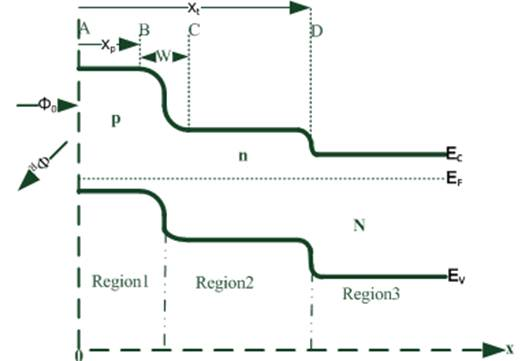Figure 1. Energy band diagram of p-n-N heterojunction grown on GaSbN substrate.

2.1.1. Calculation of the Jn Current Density of Electron Diffusion in Front Layer

This current density is obtained from the following continuity equation :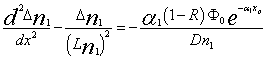(1)

where Ф0 is the incident photon flux density at the surface of the front layer (area AB Figure 1), R is the reflection coefficient at the surface of the front layer, Ln1 the electron diffusion length, α1 the absorption coefficient of the front layer, Dn1 the electron diffusion coefficient in the region1 and Δn1 the electron charge density evolution in the AB area.

The solution of this equation is in the form: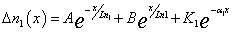(2)

With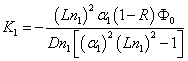(3)

The constants A and B are obtained from the following boundary conditions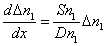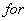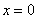(4)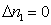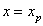(5)

The current density of electron diffusion in region 1 (zone AB Fig 1) is defined by :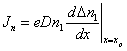(6)(7)

This photocurrent is due to the absorption of photons in the layer P of xp depth (area AB in Figure 1). This photocurrent is essentially an electron current whose the same expression is given by A. Joullie et al .

The spectral response or the internal quantum efficiency of a photodetector is defined as the ratio of the number of collected charge carriers to the number of absorbed photons. It is expressed by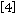,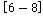: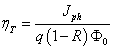(8)

where q is the elementary charge of the minority carrier, Jph the density of the total photocurrent of the detector. For each radiation, this internal quantum efficiency, results to components generated at different depths. The contribution of electrons generated in the transmitter (Figure 1 area AB), and collected by the p-n junction (Figure 1) is described by the formula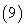.(9)

This expression is almost identical to that of Le Nam, M. Rodot and al 

2.1.2. Calculation of the Jp Hole Diffusion Current Density in Base (Region 2)

This current density is obtained from the following continuity equations :(10)(11)

where Lp2 and Lp3 are the diffusion lengths of holes, respectively in the regions 2 and 3, α2 and α3 are the absorption coefficients of the layers 2 and 3 and Dp2 and Dp3, are the diffusion coefficients of the holes in regions 2 and 3, and Δp2 and Δp3 are the evolutions of the charge density of the holes in the regions 2 and 3.

The solutions of these equations are in the form: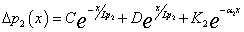(12)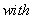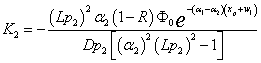(13)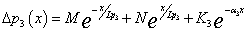(14)(15)

The constants C, D, M and N are obtained from the following boundary conditions: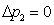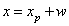(16)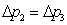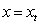(17)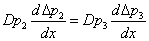(18)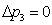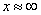(19)

The diffusion current density of the holes in region 2 (zone CD Figure 1) is defined by :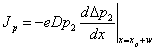(20)(21)

From equations (8) and (21), we get the expression of the internal quantum efficiency of the base (formula 22), which describes the contribution of holes generated in base (CD areaFigure1) and collected by the p-n junction:(22)

2.1.3. Calculation of the Diffusion Current Density in the Space Charge Zone (Jzce)

In the region of space charge (area BC Figure1), the electron-hole pairs are generated by the interaction with photons and dissociated by the electric field. Electrons are sent to the n region, and the holes to the p area.

At any point x of the space-charge area, there is a current density which is the sum of the electron current density generated between xp and x with the current density of holes generated between x and xp + w .

The contribution of the current density in space-charge area is given by :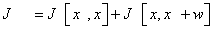(23)

In particular, at xp + w, only the electron current density must be taken into account. In the space charge zone, in stationary regime and in the absence of recombination, and to one dimension, for the electrons, the continuity equation is given by :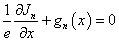(24)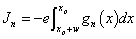(25)

After integration we get: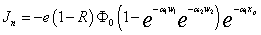(26)

we ignore the reflection at the interface (the dielectric constants of both sides of the junction are identical at the junction ).

From the equations (8) and (26), we get the expression of the internal quantum efficiency of the space charge zone (formula 27), which describes the contribution of the carriers generated in this zone (figure1) and collected by the p-n junction: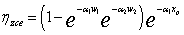(27)

2.2. Structure of a Homojunction P-p-n Grown on Substrate with Window Layer (P-Type): The Model of Ga1-yAlySbP/Ga1-xInxSbp/Ga1-xInxSbn/ GaSbN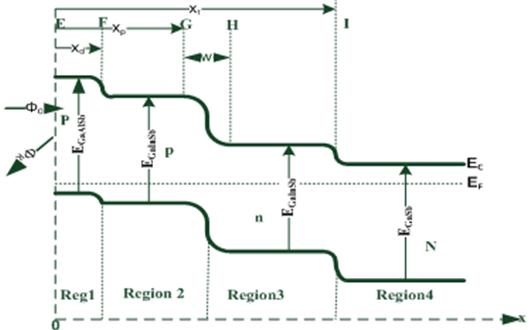Figure 2. Energy band diagram of Ga1-yAlySbP/Ga1-xInxSbp/Ga1-xInxSbn homojunction grown on GaSbN substrate.

In this case we will assume that the junction is located between the p-doped layer and the n-doped layer. The geometrical parameters are shown at Figure2. The photocurrent density in the space charge zone and the base are calculated in the same manner as in 2.1. The results differ from a pre coefficient, due to the presence of the first layer P(area EF Figure2) used as a window layer. As against, the photocurrent densities in regions 1 and 2 will change. We will consider, in the calculations of the photocurrent density in these two regions, the recombinations at surface of the first front layer and at interface of the two layers.

In the region2 (FG area Figure2), the expression of the current density resulting from the diffusion of electrons is: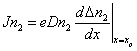(28)

This current density is obtained from the following continuity equations :(29)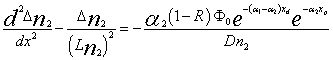(30)

where Ln1et Ln2 are the diffusion lengths of electrons, respectively in regions 1 and 2, α1 and α2 are the absorption coefficients of the layers 1 and 2, Dn1 and Dn2 the electron diffusion coefficients in regions 1 and 2, Δn1 and Δn2 are the variations of electrons charge densities in the regions 1 and 2.

The solutions of these equations are in the form:(31)(32)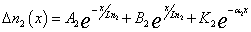(33)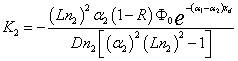(34)

The constants A1, B1, A2 and B2 are obtained from the following boundary conditions :(35)(36)(37)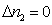(38)

The Jn2 distribution of current density of the electrons in region2 (FG area Figure2) is calculated by:(39)

From equations (8) and (39), we derive the expression of the internal quantum efficiency of the transmitter (Formula 40), which describes the contribution of electrons generated in the transmitter and in the window layer (FG and EF zones figure2) and collected by the p-n junction.(40)

3. Spectral Responses

We call spectral response, the number of charge carriers (electrons or holes) created by each photon. It depends on the wavelength and is between 0 to 1. It is also called quantum efficiency. The same information is provided by the determination of the spectral sensitivity, which is expressed in A/W.

Theoretical models are applied to various devices corresponding to best photodetectors in the range of wavelength 0,78μm, to 2,6μm. We report the best results of the influence of photoelectric parameters (diffusion lengths and recombination velocity) and geometrical parameters (thickness of the transmitter and window layer) on the internal quantum efficiency or sensitivity given by each case of the used theoretical model. The work is devoted on the heterostructures based on Ga1-xInxSband Ga1-yAlySb (x = 0.18, y = 0.08) deposited on GaSbN substrate.

3.1. Influence of the Electron Recombination Velocity at the Transmitter Surface (The Models Ga1-xInxSbp/Ga1-xInxSbn/GaSbN and, Ga1-y AlySbp/ Ga1-xInxSbn /GaSbN)

The figure3.a and figure3.b show the influence of the recombination velocity at surface on the internal quantum efficiency versus photon energy for a Ga1-xInxSbp/Ga1-xInxSbn/GaSbN homojunction (figure 3a) and a Ga1-yAlySbp/Ga1-xInxSbn/GaSbN heterojunction (figure 3b).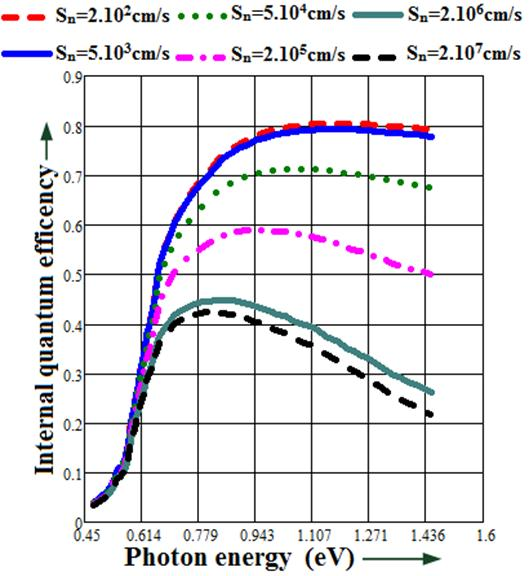Figure 3a). Quantum efficiency for different recombination velocities values vs. photon energy of Ga1-xInxSbp/Ga1-xInxSbn/GaSbN homojunction (Ln1=0.5µm, Lp2=5µm, Lp3=6µm, xp=1.2µm, xt=7µm, w=0.7µm).

There is a strong decrease in the internal quantum efficiency with increasing the recombination velocity for Sn values ranging from 5.103cm/s to 2.106cm/s(Figure3). Below 5.103cm/s and, beyond 2.106cm/s, the recombinations at surface have practically no influence on the quantum efficiency. This phenomenon is related to the fact that the recombination effect at surface is less preponderant on the recombination effect in volume, whose recombination velocity is of the order of Dn/Ln .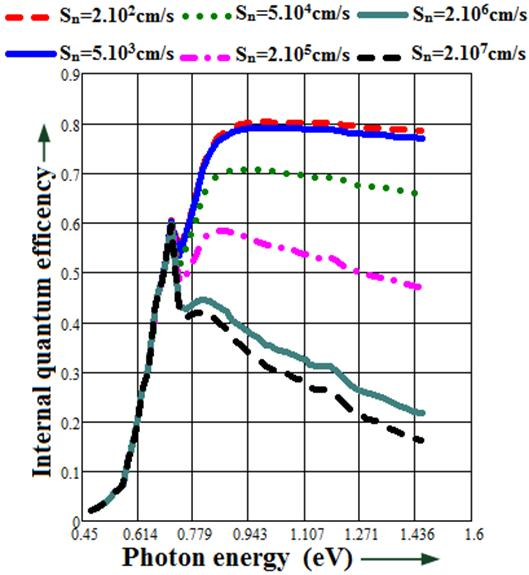Figure 3b). Quantum efficiency for different recombination velocities values vs. photon energy of Ga1-yAlySbP/ Ga1-xInxSbn/ GaSbN heterojunction (Ln1=0.5µm, Lp2=5µm, Lp3=6µm, xp=1.2µm, xt=7µm, w=0.7µm).

This result gives us information about the possible limits of the recombination velocities at surface and at interface for these models of devices. On figure3b, we observe two peaks, of which the first corresponds to the absorption in the base and the second that of the transmitter.

Indeed, the Ga1-yAlySbp material, having higher gap energy than that of the Ga1-xInxSbn, remains transparent to energy values less than its gap energy, allowing thereby the Ga1-xInxSbn material to absorb at first. The decrease in quantum efficiency, just after the maximum of the second peak, is related to recombination phenomenon at surface that prevent a portion of the electrons generated in the vicinity of the surface of the transmitter to contribute to the photocurrent. This recombination phenomenon at surface is due to a large number of the recombinant centers, to an important density of states of interfaces related to defects of structure and impurities.

3.2. Influence of the Transmitter Thickness on the Quantum Efficiency (The Models Ga1-xInxSbp/Ga1-xInxSbn/GaSbN and, Ga1-yAlySbp/ Ga1-xInxSbn/GaSbN)

The figure4.a and figure4.b show the influence of the thickness of the transmitter on the internal quantum efficiency versus photon energy for a Ga1-xInxSbp/Ga1-xInxSbn/GaSbN homojunction (figure4a) and a Ga1-yAlySbp/Ga1-xInxSbn/GaSbN heterojunction (figure4b).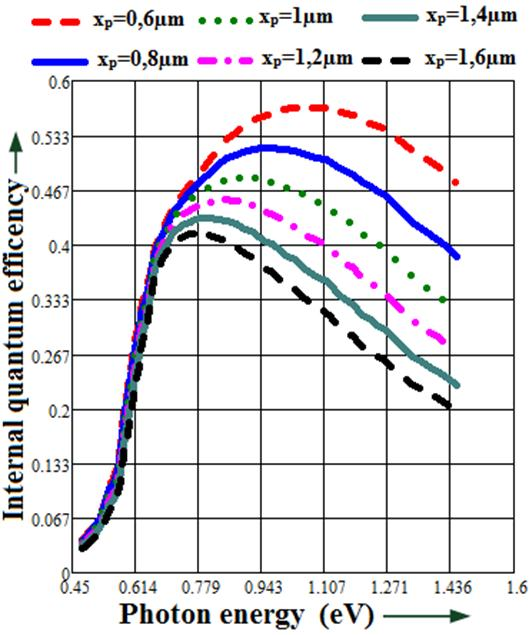Figure 4a. Quantum efficiency for different values of the thickness vs. photon energy of Ga1-yAlySbP/Ga1-xInxSbp/Ga1-xInxSbn/GaSbN heterojunction (Sn1=2.106cm/s, Ln1=0.5µm, Lp2=5µm, Lp3=6µm, xt=7µm, w=0.7µm).Figure 4b. Quantum efficiency for different values of the thickness vs. photon energy of Ga1-yAlySbP/Ga1-xInxSbp/Ga1-xInxSbn/GaSbN homojunction (Sn1=2.106cm/s, Ln1=0.5µm, Lp2=5µm, Lp3=6µm, xt=7µm, w=0.7µm).

Whatever model considered, an increase in signal is observed when we decreases the transmitter thickness. However, we note that for low thicknesses, the maximum is obtained, to high energies for the homojunction (figure4a) while for the heterojunction model (figure4b), the maximums are obtained with low energies, corresponding to wavelength of about 1,75μm.

For Ga1-xInxSbp/Ga1-xInxSbn/GaSbN model, this decrease of quantum efficiency is related to the fact that at high thicknesses, only the carriers (electrons) generated near the junction, manage to achieve the junction and to contribute to the photocurrent, while for low thicknesses, most of the carriers generated near the surface contribute to the photocurrent. The phenomenon observed in the model Ga1-yAlySbp/Ga1-xInxSbn/GaSbN is due to the fact that the Ga1-yAlySbp layer remains transparent to low energies allowing thereby, a strong generation of holes at grassroots level and which will contribute to the photocurrent, while in the model Ga1-xInxSbp/Ga1-xInxSbn/GaSbN few photons achieve the base. The slight decrease in signal, observed immediately after the maximum, is attributed to recombination phenomenons.

3.3. Influence of the Widow Layer Thickness on the Sensitivity (The Model Ga1-yAlySbP/Ga1-XInXSbp/Ga1-xInxSbn/GaSbN)

Figure 5 shows the influence of the window layer thickness on the sensitivity versus photon energy for a Ga1-yAlySbP/Ga1-XInXSbp/Ga1-xInxSbn/GaSbN homojunction.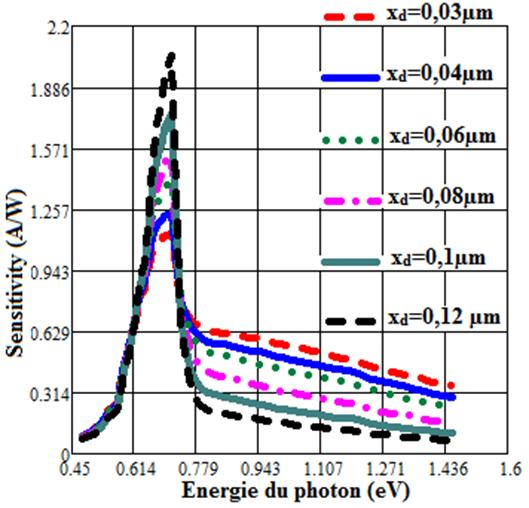Figure 5. Sensitivity for different values of the window layer thickness vs. photon energy of Ga1-yAlySbP/Ga1-xInxSbp/Ga1-xInxSbn/GaSbN homojonction (Sn1=2.106cm/s, Sn2=2.103cm/s, Ln1=0.5µm,Ln2=1µm, Lp3=5µm, Lp4=6µm, xp=0.4µm, xt=7µm, w=0.7µm).

The sensitivity analysis for different values of the thickness of the window layer shows a net improvement in the sensitivity of the device with the increase in the thickness of the window layer. However, this increase suggests a small value of the thickness of the emitter (xp=0,4µm Figure 5). It is possible to achieve sensitivity of the order of 2.05A /W for a thickness of the window layer of the order of 0.12μm and that of the emitter of the order of 0.28μm. For these thickness values, the internal quantum efficiency is of the order of 96% for photon energy of 0.72eV either in the absorption spectrum of the near infrared. Indeed, the window layer remains transparent to the energy photon flux lower than its bandgap energy, whereas the low value of the emitter thickness leads to a low absorption in the emitter, which allows to the maximum of photons to be absorbed in the base, and in the space charge area. The photons arriving in the base and in the space charge area generate free carriers, which in turn, contribute to the photocurrent, resulting in a net improvement of the sensitivity of the device.

3.4. Comparative Study of the Sensitivity of Different Models of Photodetectors Versus the Radiation Energy

Figure6 shows the sensitivity of photodetectors devices versus the energy of the radiation. The rising edge of the sensitivity curves is abrupt. This result is due to the rapid increase of the absorption coefficient with the photon energy, typical of a direct gap material.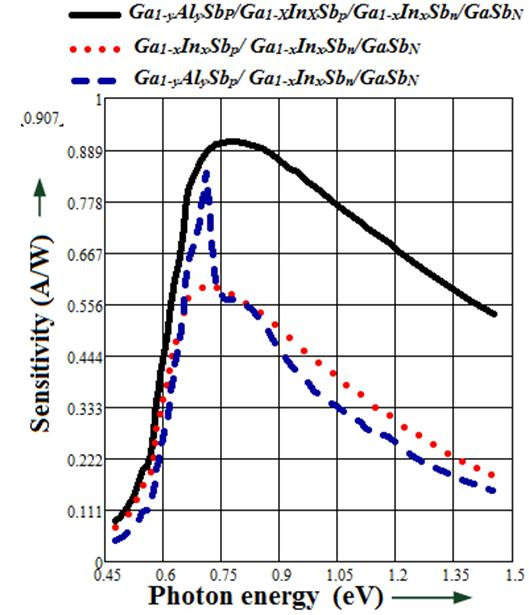Figure 6 .Sensitivity of photodetectors devices versus the energy of the radiation (Sn1=2.106cm/s, Sn2=2.103cm/s, Ln1=0.5µm,Ln2=1µm, Lp3=5µm, Lp4=6µm,xd=0.1µm, xp=1.2µm, xt=7µm, w=0.7µm).

The decrease of the signal observed immediately after the maximum, is attributed to recombination phenomenons at surface. We note that the position of the maximum of the sensitivity of the photodetectors depends on the model; that is to say, at the transition energy of bandgap of the layers which compose them.

This position of the maximum, lies practically at an energy E0= 0,71eV, corresponding to a wavelength λmax = 1,75μm slightly less than the cutoff wavelength defined by λc (μm) = 1.24/E0 (eV). The study of the sensitivity of the photodetectors models shows that the Ga1-yAlySbP/Ga1-xInxSbp/Ga1-xInxSbn/GaSbN model has a maximum sensitivity of about 0,907A/W substantially greater than that of Ga1-xInxSbp/Ga1-xInxSbn/GaSbN (0,593A/W) and the Ga1-yAlySbP/Ga1-xInxSbn/GaSbN (0,84A/W). This difference is related to the presence of the Ga1-yAlySbP window layer, which in the first time, remains transparent to low energies, for better absorption in the transmitter and in the base. It plays an important role in the reduction of recombinations at surface and at the interface thus allowing a maximum of minority carriers to be collected.

4. Conclusion

In this article, we analyzed the performance of photoelectric detectors corresponding to three different models: a Ga1-xInxSbp/Ga1-xInxSbn/GaSbN homojunction and a Ga1-yAlySbp/Ga1-xInxSbn/GaSbN heterojunction deposited on the GaSbN substrate, and a Ga1-yAlySbP/Ga1-XInXSbp/Ga1-xInxSbn/GaSbN homojunction deposited on the GaSbN substrate, with Ga1-yAlySbP as window layer. This analysis is based on the photon energy of the incident light, and the photoelectric and geometrical parameters of the various devices. In each model, we determined the internal quantum efficiency that we simulated to appreciate the photoelectric behavior of the different models. Theoretical analysis of the spectral response of these photodetectors models has allowed us to understand their conduction mechanisms and the limits of their performances. The improvement of the spectral response requires the use of a thin transmitter. However, we note that to obtain a high internal quantum efficiency at low energies (wavelengths between 0,78μm to 2,6μmeither in the absorption spectrum of the near infrared), it is necessary to use average thicknesses around 1,2μm.Analysis of the results shows that the sensitivity is much important, with the low incidences, through a Ga1-yAlySbp/Ga1-xInxSbn/GaSbN heterojunction than through a Ga1-xInxSbp/Ga1-xInxSbn/GaSbN homojunction for both structures. The recombinations at surface and at volume significantly limit the performances of Ga1-xInxSbp/Ga1-xInxSbn and Ga1-yAlySbp/Ga1-xInxSbn deposited on the GaSbN substrate. The window layer of the Ga1-yAlySbP/Ga1-xInxSbp/Ga1-xInxSbn/GaSbN plays an important role in reducing these recombinations allowing thereby to get a better sensitivity around 0.907 A/W (Ln =1µm, xp=1.2µm and xd=0.1µm). Ultimately, the performance of an infrared photodetector device, beyond its photoelectric and geometrical parameters, depends on the corresponding model and consequently the Ga1-yAlySbP/Ga1-XInXSbp/Ga1-xInxSbn homojunction grown on GaSbN substrate is the model allowing to obtain better detection in the range between 0,78μm to 2,6μm (absorption spectrum of the near infrared).

References

1. J. Chen, Y. Zhou, Z. Xu, J. Xu, Q. Xu, H. Chen, L. He. InAs/GaSb type-II superlatice mid-wavelength infrared focal plane array detectors grown by molecular beam epitaxy. Journal of Crystal Growth 378, 596-599, (2013)
2. L. Gouskov, M. Boustani, G. Bougnot, C. Gril, A. Joullie et al.Photodétecteurs à base de Ga1-xAlxSb dans la gamme 1,3-1,6µm. Revue de physique Appliquée, 18(12), 781-782, (1983).
3. P. Viktorovitch. Passivation des sémiconducteurs III-V.Revue Phys.Appl 25 895-914, (1990).
4. B. MBOW, A. MEZERREG, N. REZZOUG, and C. LLINARES. Spectral Responses in Near-infrared of III-V Photodetectors. Phys, sat. sol (a) 141, 511-525, (1994).
5. YUAN. TIAN et al. The analysis of the performance for Photodetectors. Phys, sat. sol(a) 174, 414, (1999).
6. A Joullié, F. De Anda, P. Salsac. Diffusion du zinc dans GaAlSb et application à la photodétection infrarouge.Revue Phys. Appl. 19223-230 (1984).
7. C. Touzi, Z. Chine, T. Boufaden, B. El Jani. Etude de la réponse spectacle des cellules photovoltaïques à base de GaN.Phys, Chem.News 1 69-72, (2001).
8. S. N. SINGH and P. K. SINGH. Exact calculation of back surface recombination velocity and its influence on quantum efficiency of n+-p-p+ structure based silicon cells. Proc IEEE 1629-1631, (1988).
9. L. Nam, M. Rodot et al. Réponse spectacle de photopiles de haut rendement au silicium multicristallin.Journal de physique III EDP Sciences, 2 (7), 1305-1316, (1992).
10. H Mathieu & H Fanet.Physique des sémiconducteurs et des composants électroniques, Dunod: 6ème édition
11. J. Bozec et G. Rolland. Evaluation de la longueur de diffusion par la méthode EBIC dans les piles solaires à l’AsGa et application à l’environnement spatial.Revue de Physique Appliquée, 21 (8), 509-514, (1986).

 Contents 1. 2. 2.1. 2.2. 3. 3.1. 3.2. 3.3. 3.4. 4.
Article ToolsAbstractPDF(584K)